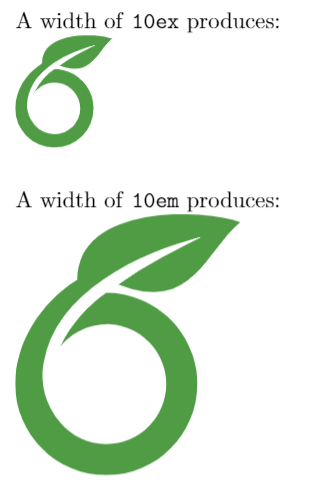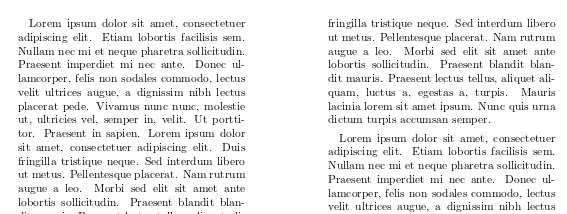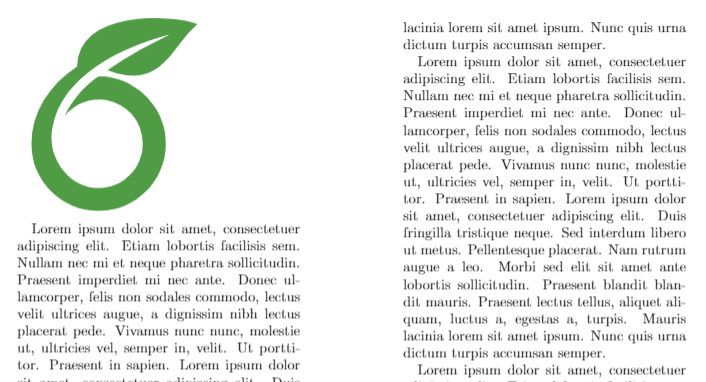Contents 1 Units 2 Lengths 3 Using lengths as units 4 Further reading In LaTeX there are a lot of lengths determining various dimensions of prepared documents. For example, specified dimension parameters characterize fonts, pages, or paragraphs. Units Below a description of available units in LaTeX. Abbreviation Value pt a point is approximately 1/72.27 inch, that means about 0.0138 inch or 0.3515 mm (exactly point is defined as 1/864 of American printer’s foot that is 249/250 of English foot) mm a millimeter cm a centimeter in inch ex roughly the height of an 'x' (lowercase) in the current font (it depends on the font used) em roughly the width of an 'M' (uppercase) in the current font (it depends on the font used) mu math unit equal to 1/18 em, where em is taken from the math symbols family sp so-called "special points", a low-level unit of measure where 65536sp=1pt Below an example that shows the difference between ex and em units. \documentclass[twocolumn]{article} \usepackage{blindtext} \usepackage{graphicx} \setlength{\columnsep}{1in} \begin{document} A width of \texttt{10ex} produces: \includegraphics[width=10ex]{overleaf-logo} \vspace{5mm} A width of \texttt{10em} produces: \includegraphics[width=10em]{overleaf-logo} \end{document}Open an example in Overleaf Lengths Lengths are units of distance relative to some document elements. Lengths can be changed by the command: \setlength{\lengthname}{value_in_specified_unit} For example, in a two-column document the column separation can be set to 1 inch by: \setlength{\columnsep}{1in}Below is a table with some of the most common lengths and their description Length Description \baselineskip Vertical distance between lines in a paragraph \columnsep Distance between columns \columnwidth The width of a column \evensidemargin Margin of even pages, commonly used in two-sided documents such as books \linewidth Width of the line in the current environment. \oddsidemargin Margin of odd pages, commonly used in two-sided documents such as books \paperwidth Width of the page \paperheight Height of the page \parindent Paragraph indentation \parskip Vertical space between paragraphs \tabcolsep Separation between columns in a table (tabular environment) \textheight Height of the text area in the page \textwidth Width of the text area in the page \topmargin Length of the top margin  Open an example in Overleaf Using lengths as units Default lengths can be set to any desired value up to a maximum of approximately 16384pt (or 5.75 metres), a hard-coded limit built into TeX engines. LaTeX's length parameters can also be used as units to set the dimensions of other LaTeX elements. For instance, you can set an image to have a width of one quarter the total text width: \includegraphics[width=0.25\textwidth]{overleaf-logo} [...]In the command \includegraphics the width is set to 0.25 the width of the entire text area (see Inserting Images for more information about this command). You can use any length and multiply it by any factor.  Open an example in Overleaf Further reading For more information see the article about lengths on wikibooks.